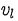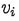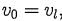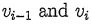106 年 - 106 國立中山大學_碩士班招生考試_資工系(甲組)：離散數學#105791

【非選題】
1.
There are 7 problems in this test. No calculators are allowed. Write down detailed steps for the solution to each problem. Otherwise, no credits for that problem will be given.

【題組】

1. Let x1, x2, ,be a sequence of n integers. A consecutive subsequence of x1,x2,... .,is a subsequencefor some i, j, 1 ≤ i ≤ j ≤n. Show that for any k, I k n, there is a consecutive subsequence whose sum is divisible by k.

【非選題】
2.【題組】

2. Assume that a sequence of numbers is deined by x0 = 0, x1 = 1, and=＞ 1. Find generating function for the sequence, and then find an explicit expression for un.

【非選題】
3.【題組】

3. Show thatGive combinatorial explanation to the equation.

【非選題】
4.

There are 7 problems in this test. No calculators are allowed. Write down detailed steps for
the solution to each problem. Otherwise, no credits for that problem will be given.
4. A bipartite graph is a graph whose vertices can be partitioned into two subsets X and Y so that each edge has one end in X and the other end in Y: A cycle of G is a sequence of vertices u0, u1, ...,such that each vertexis distinct, except,and； are adjacent for each i = 1,2,. ,I. The length of the cycle is l. A k-cube is a graph whose vertices are binary strings of length k, for some integer k ＞ 0.Two vertices are adjacent if and only if they differ in exactly one bit.

【題組】(a) Show that every h-cube is bipartite by partitioning its vertexes into X and Y, and then show that every edge connects some vertex in X and another vertex in Y.

【非選題】
5.【題組】(b) Show that a bipartite graph has no cycles of odd length.

【非選題】
6.【題組】(c) Show that if a graph has no cycles of odd length then it is bipartite.

【非選題】
7.
There are 7 problems in this test. No calculators are allowed. Write down detailed steps for the solution to each problem. Otherwise, no credits for that problem will be given.

【題組】5. Suppose that 5 points are chosen in a square whose sides have length 2. Show that there must be at least two points p and g such that the distance between them is no more than √2.

【非選題】
8.【題組】

6. Fibonacci numbers are deined as f0 = 0, f1= 1 andfor n ＞.1. Show thatis even, for every positive integer k. .

【非選題】
9.
There are 7 problems in this test. No calculators are allowed. Write down detailed steps for the solution to each problem. Otherwise, no credits for that problem will be given.
7. Let m and n be two positive integers, m ≤ n. Define【題組】

(a) Show that if'n is prime, then n dividesfor every i, 1 ≤i＜n.

【非選題】
10.【題組】

(b) Show that if n is composite, then n does not dividefor some i, 1 ≤i ＜n.

懸賞詳解

國二國文下第三次

【已刪除】4.( ) 下列是陳極渲的姪子寄給他的信，請問信封的書 寫，哪裡「錯誤」？ (A)郵票貼處(B)啟封詞(C)寄信人住址 (D...